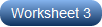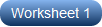# Solving Quadratic Equations by Completing the Squares Worksheets

Transform any quadratic equation that cannot be factored to the one that can be factored, with this simple never-fail technique of completing squares. Bolster practice using these printable worksheets on solving quadratic equations by completing the squares, and solve the trickiest of quadratic equations effortlessly. High school students begin by moving the constant to the right side and rearranging the left side of the equation to make it a complete square of the form x2+ 2ax+ a2. Divide the coefficient of x by 2 and square it to find the value of a2. Balance the equation by adding the value of a2 on either side of the equation to make a complete square (x+a)2. The stage is now set to solve the quadratic equation by taking the square root on both sides and computing to find the roots of the quadratic equation. Our free worksheet will leave you yearning for more!

Leave no stone unturned in learning this technique of completing squares to solve quadratics. These easy level pdf worksheets comprise equations with no coefficient for x2. Students need to follow the sequence of steps meticulously and that's mission accomplished!Things get a little trickier as you move up the ladder. The quadratic equations in these printable worksheets have coefficients for the term x2 that need to be factored out. Divide the entire equation by the coefficient of x2, apply the series of steps to complete the squares, and solve.High school students often get intimidated by the fractions, decimals, and the square roots in the quadratic equations. Remember, there's always a way out! Devising and applying strategies like the substitution method make the process of solving quadratic equations by completing squares a cakewalk.